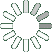Puzzle Command
Daily Puzzle
Number Logic #15
February 8, 2015Find a 6 digit number where:

The 1st digit is equal to 9.
The 2nd digit is the number of 5s and is odd.
The 3rd digit is equal to 1.
The 4th digit is equal to 9.
The 5th digit is the number of 9s.
The 6th digit is odd and a prime number.

Puzzle Notes
6 digit numbers range from 100,000 to 999,999.
Enter in your solution below and click submit.